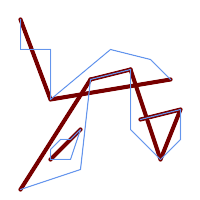## Name

ST_Simplify — Returns a simplified representation of a geometry, using the Douglas-Peucker algorithm.

## Synopsis

`geometry ST_Simplify(`geometry geom, float tolerance`)`;

`geometry ST_Simplify(`geometry geom, float tolerance, boolean preserveCollapsed`)`;

## Descripción

Computes a simplified representation of a geometry using the Douglas-Peucker algorithm. The simplification `tolerance` is a distance value, in the units of the input SRS. Simplification removes vertices which are within the tolerance distance of the simplified linework. The result may not be valid even if the input is.

The function can be called with any kind of geometry (including GeometryCollections), but only line and polygon elements are simplified. Endpoints of linear geometry are preserved.

The `preserveCollapsed` flag retains small geometries that would otherwise be removed at the given tolerance. For example, if a 1m long line is simplified with a 10m tolerance, when `preserveCollapsed` is true the line will not disappear. This flag is useful for rendering purposes, to prevent very small features disappearing from a map.The returned geometry may lose its simplicity (see ST_IsSimple), topology may not be preserved, and polygonal results may be invalid (see ST_IsValid). Use ST_SimplifyPreserveTopology to preserve topology and ensure validity.This function does not preserve boundaries shared between polygons. Use ST_CoverageSimplify if this is required.

## Ejemplos

A circle simplified too much becomes a triangle, medium an octagon,

```SELECT ST_Npoints(geom) AS np_before,
ST_NPoints(ST_Simplify(geom, 0.5)) AS np05_notquitecircle,
ST_NPoints(ST_Simplify(geom, 1)) AS np1_octagon,
ST_NPoints(ST_Simplify(geom, 10)) AS np10_triangle,
(ST_Simplify(geom, 100) is null) AS  np100_geometrygoesaway
FROM (SELECT ST_Buffer('POINT(1 3)', 10,12) As geom) AS t;

np_before | np01_notbadcircle | np05_notquitecircle | np1_octagon | np10_triangle | np100_geometrygoesaway
-----------+-------------------+---------------------+-------------+---------------+------------------------
49 |                33 |                  17 |           9 |             4 | t
```

Simplifying a set of lines. Lines may intersect after simplification.```SELECT ST_Simplify(
'MULTILINESTRING ((20 180, 20 150, 50 150, 50 100, 110 150, 150 140, 170 120), (20 10, 80 30, 90 120), (90 120, 130 130), (130 130, 130 70, 160 40, 180 60, 180 90, 140 80), (50 40, 70 40, 80 70, 70 60, 60 60, 50 50, 50 40))',
40);
```

Simplifying a MultiPolygon. Polygonal results may be invalid.```SELECT ST_Simplify(
'MULTIPOLYGON (((90 110, 80 180, 50 160, 10 170, 10 140, 20 110, 90 110)), ((40 80, 100 100, 120 160, 170 180, 190 70, 140 10, 110 40, 60 40, 40 80), (180 70, 170 110, 142.5 128.5, 128.5 77.5, 90 60, 180 70)))',
40);
```